School of Economics and Management
Beihang University
http://yanfei.site

## Packages required in this lecture

• caret and its dependences

## Unsupervised methods

### Clustering: working without known targets

• Suppose you want to segment your customers into general categories of people with similar buying patterns.
• You might not know in advance what these groups should be.

## Supervised methods

### Classification: deciding how to assign (known) labels to an object.

• Supervised learning
• To learn how to classify objects, you need a dataset of objects that have already been classified (training set)

## Examples of classification problems

• Identifying spam email
• Sorting products in a product catalog
• Identifying loans that are about to default
• Assigning customers to customer clusters

## Some common classification methods

### Logistic regression

• Logistic regression is appropriate when you want to estimate class probabilities (the probability that an object is in a given class).

• An example use of a logistic regression–based classifier is estimating the probability of fraud in credit card purchases.

• Logistic regression is also a good choice when you want an idea of the relative impact of different input variables on the output. For example, you might find out that a $100 increase in transaction size increases the odds that the transaction is fraud by 2%, all else being equal. ## Some common classification methods ### K nearest neighbours (KNN) • In k-NN classification, the output is a class membership. An object is classified by a majority vote of its neighbors, with the object being assigned to the class most common among its k nearest neighbors (k is a positive integer, typically small). • If k = 1, then the object is simply assigned to the class of that single nearest neighbor. ## Some common classification methods ### Naive Bayes Naive Bayes classifiers are especially useful for problems with many input variables, categorical input variables with a very large number of possible values, and text classification. ## Some common classification methods ### Decision trees • Decision trees are useful when input variables interact with the output in “if-then”" kinds of ways (such as IF age > 65, THEN has.health.insurance=T). • They are also suitable when inputs have an AND relationship to each other (such as IF age < 25 AND student=T, THEN…) or when input variables are redundant or correlated. • The decision rules that come from a decision tree are in principle easier for nontechnical users to understand than the decision processes that come from other classifiers. • Random forests are important extensions of decision trees. ## Some common classification methods ### Support vector machines • Support vector machines (SVMs) are useful when there are very many input variables or when input variables interact with the outcome or with each other in complicated (nonlinear) ways. • SVMs make fewer assumptions about variable distribution than many other methods, which makes them especially useful when the training data isn’t completely representative of the way the data is distributed in production. ## Case study: Titanic disaster • The sinking of the Titanic is one of the most infamous shipwrecks in history. One of the reasons that the shipwreck led to such loss of life was that there were not enough lifeboats for the passengers and crew. • Although there was some element of luck involved in surviving the sinking, some groups of people were more likely to survive than others, such as women, children, and the upper-class. ## Our data Each record in the dataset describes a passenger. The attributes are defined as follows: 1. PassengerId: Unique passenger identification number 2. Survived: Did a passenger survive or not (0 = died, 1 = survived) 3. Pclass: Passenger Class (1 = 1st; 2 = 2nd; 3 = 3rd) 4. Name: Name of Passenger 5. Sex: Sex of Passenger 6. Age: Passenger Age 7. SibSp: Number of Siblings/Spouses Aboard 8. Parch: Number of Parents/Children Aboard 9. Ticket: Ticket Number 10. Fare: Passenger Fare 11. Cabin: Cabin 12. Embarked: Port of Embarkation (C = Cherbourg; Q = Queenstown; S = Southampton) ## Data preparation library(caret) ## load the CSV file from the local directory datasetTrain <- read.csv("https://yanfei.site/docs/dpsa/train.csv", header=TRUE, sep = ",") datasetTest <- read.csv("https://yanfei.site/docs/dpsa/test.csv", header=TRUE, sep = ",") Our problem to solve is to predict the survival of the passengers in the test dataset. ## Data summarization ## dimension dim(datasetTrain) ##  891 12 ## attribute class type sapply(datasetTrain, class) ## ID Survived Pclass Name Sex Age SibSp ## "integer" "integer" "integer" "factor" "factor" "numeric" "integer" ## Parch Ticket Fare Cabin Embarked ## "integer" "factor" "numeric" "factor" "factor" head(datasetTrain) ## ID Survived Pclass Name ## 1 1 0 3 Braund, Mr. Owen Harris ## 2 2 1 1 Cumings, Mrs. John Bradley (Florence Briggs Thayer) ## 3 3 1 3 Heikkinen, Miss. Laina ## 4 4 1 1 Futrelle, Mrs. Jacques Heath (Lily May Peel) ## 5 5 0 3 Allen, Mr. William Henry ## 6 6 0 3 Moran, Mr. James ## Sex Age SibSp Parch Ticket Fare Cabin Embarked ## 1 male 22 1 0 A/5 21171 7.2500 S ## 2 female 38 1 0 PC 17599 71.2833 C85 C ## 3 female 26 0 0 STON/O2. 3101282 7.9250 S ## 4 female 35 1 0 113803 53.1000 C123 S ## 5 male 35 0 0 373450 8.0500 S ## 6 male NA 0 0 330877 8.4583 Q ## remove redundant variables datasetTrain <- datasetTrain[, c(-1, -4, -9, -11, -12)] ## summary summary(datasetTrain) ## Survived Pclass Sex Age ## Min. :0.0000 Min. :1.000 female:314 Min. : 0.42 ## 1st Qu.:0.0000 1st Qu.:2.000 male :577 1st Qu.:20.12 ## Median :0.0000 Median :3.000 Median :28.00 ## Mean :0.3838 Mean :2.309 Mean :29.70 ## 3rd Qu.:1.0000 3rd Qu.:3.000 3rd Qu.:38.00 ## Max. :1.0000 Max. :3.000 Max. :80.00 ## NA's :177 ## SibSp Parch Fare ## Min. :0.000 Min. :0.0000 Min. : 0.00 ## 1st Qu.:0.000 1st Qu.:0.0000 1st Qu.: 7.91 ## Median :0.000 Median :0.0000 Median : 14.45 ## Mean :0.523 Mean :0.3816 Mean : 32.20 ## 3rd Qu.:1.000 3rd Qu.:0.0000 3rd Qu.: 31.00 ## Max. :8.000 Max. :6.0000 Max. :512.33 ##  ## Percentage of survived table(datasetTrain$Survived)
##
##   0   1
## 549 342
prop.table(table(datasetTrain$Survived)) ## ## 0 1 ## 0.6161616 0.3838384 ## convert survived to factor datasetTrain[,1] <- as.factor((datasetTrain[,1])) data <- datasetTrain data[,3] <- as.numeric((data[,3])) complete_cases <- complete.cases(data) ## Data visualization ## survival bar chart ggplot(datasetTrain, aes(x = Survived)) + geom_bar() + ggtitle("Survived Bar Chart")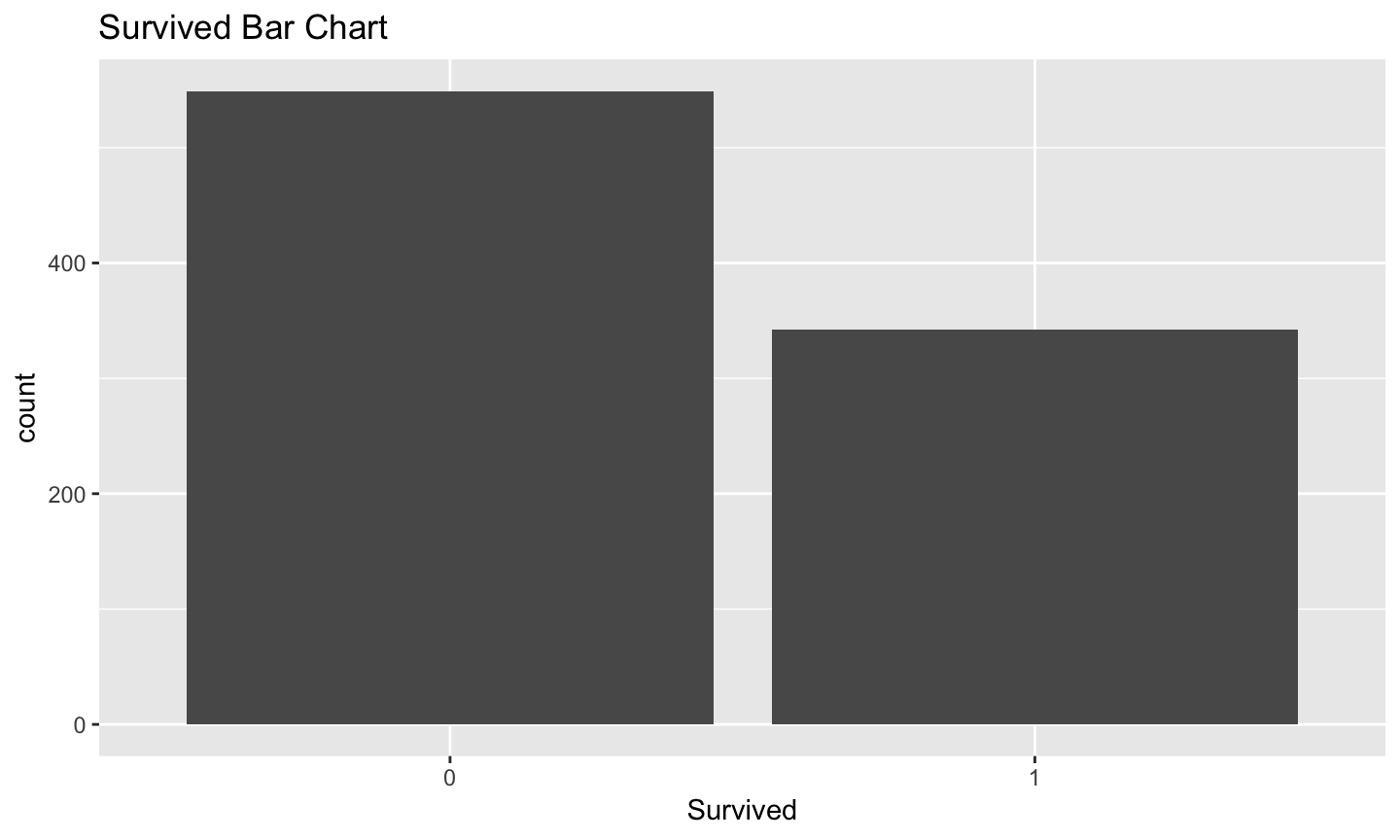## Pclass distribution ggplot(datasetTrain, aes(x = Pclass)) + geom_bar() + ggtitle("Survived Bar Chart")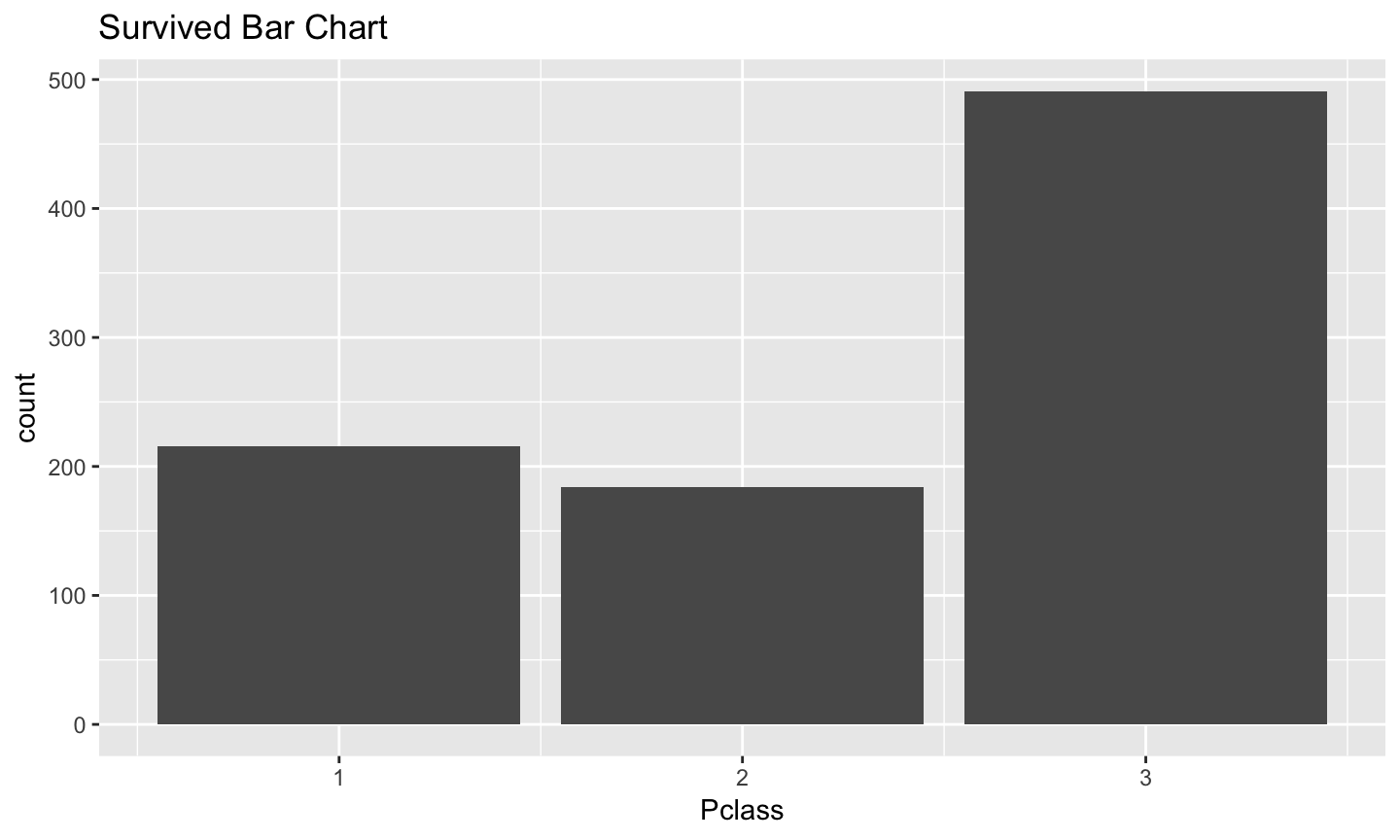## barplot of males and females in each class ggplot(datasetTrain, aes(x = factor(Pclass), fill = factor(Sex))) + geom_bar(position = "dodge")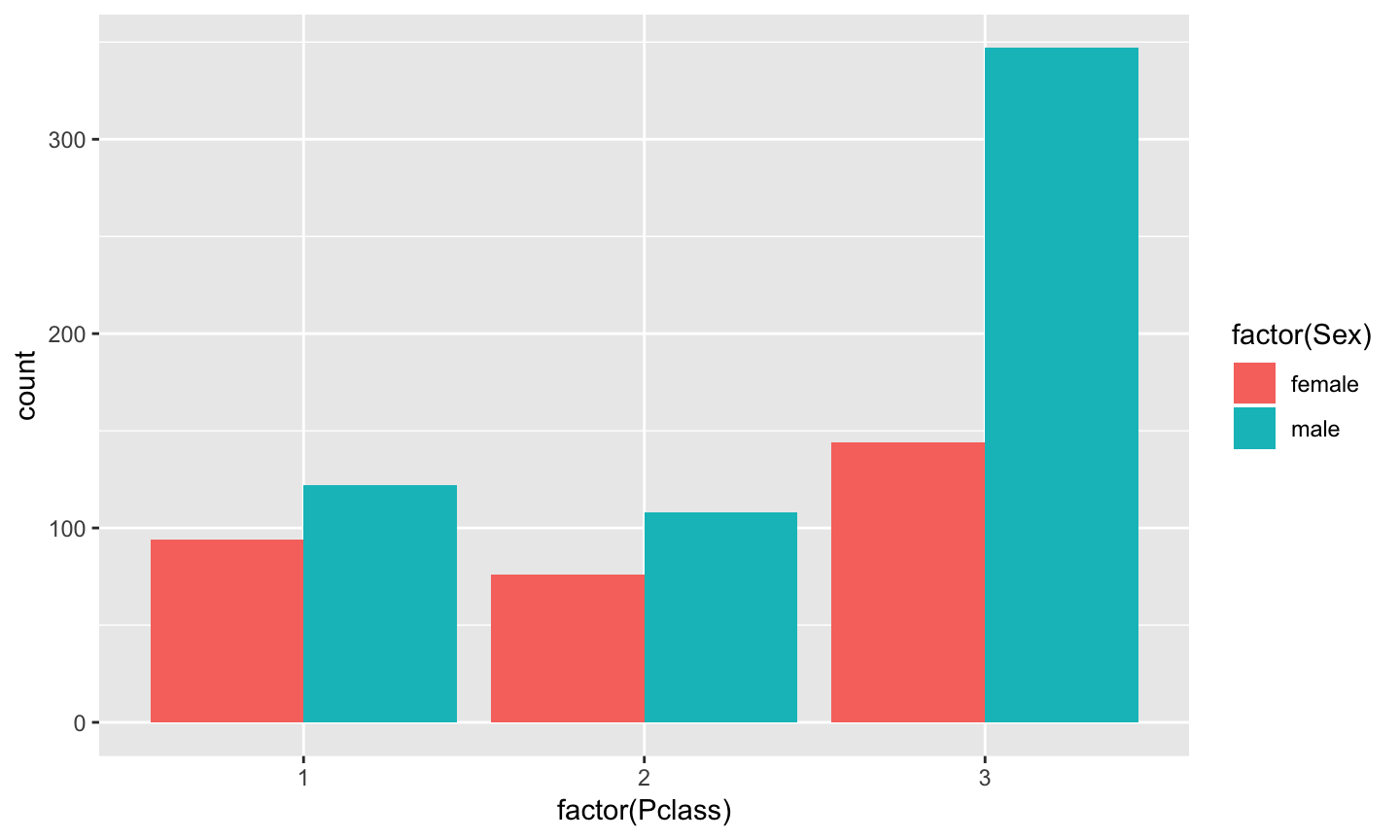## barplot of males and females who survived in each class ggplot(datasetTrain, aes(x = factor(Pclass), fill = factor(Sex))) + geom_bar(position = "dodge") + facet_grid(". ~ Survived")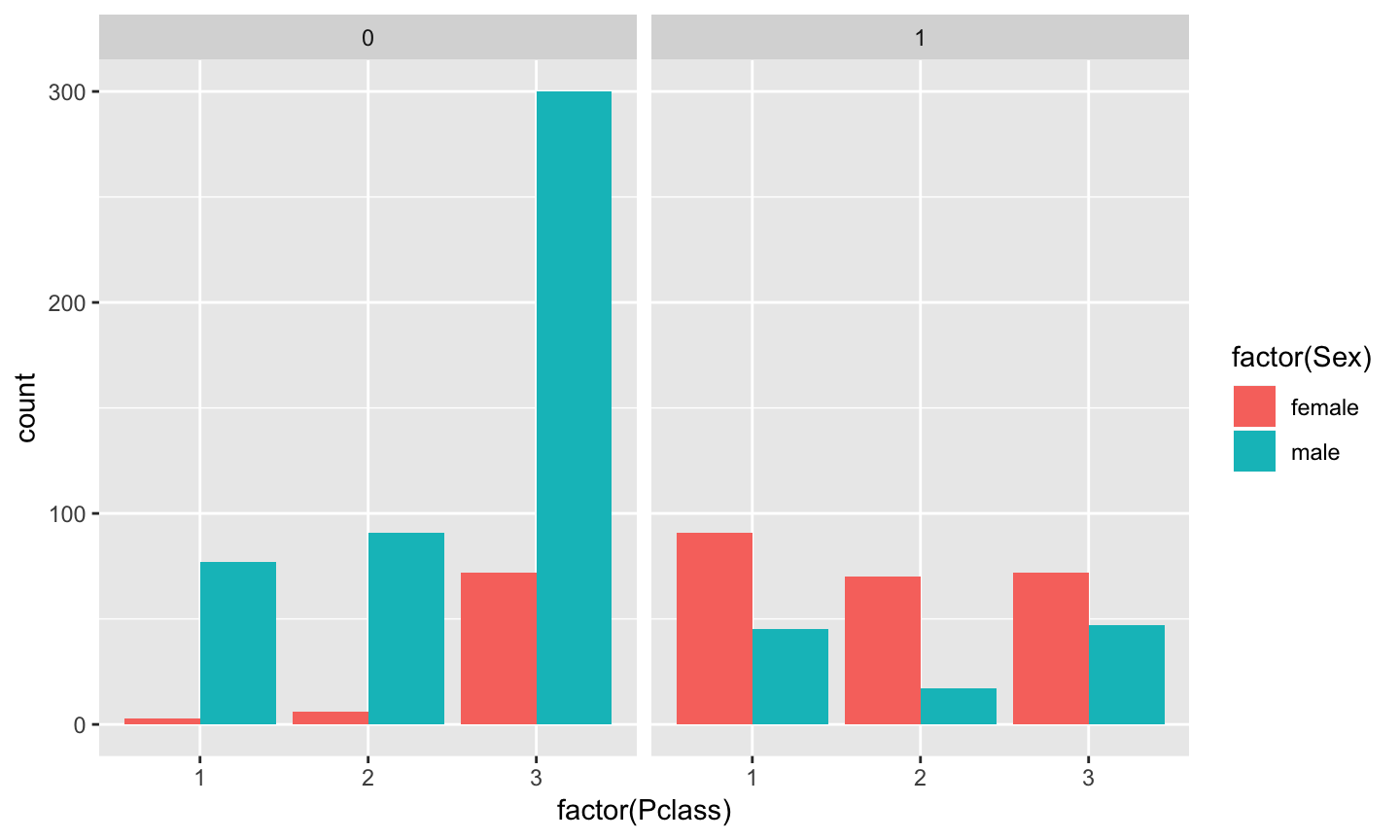library(corrplot) corrplot(cor(data[complete_cases, 2:5]))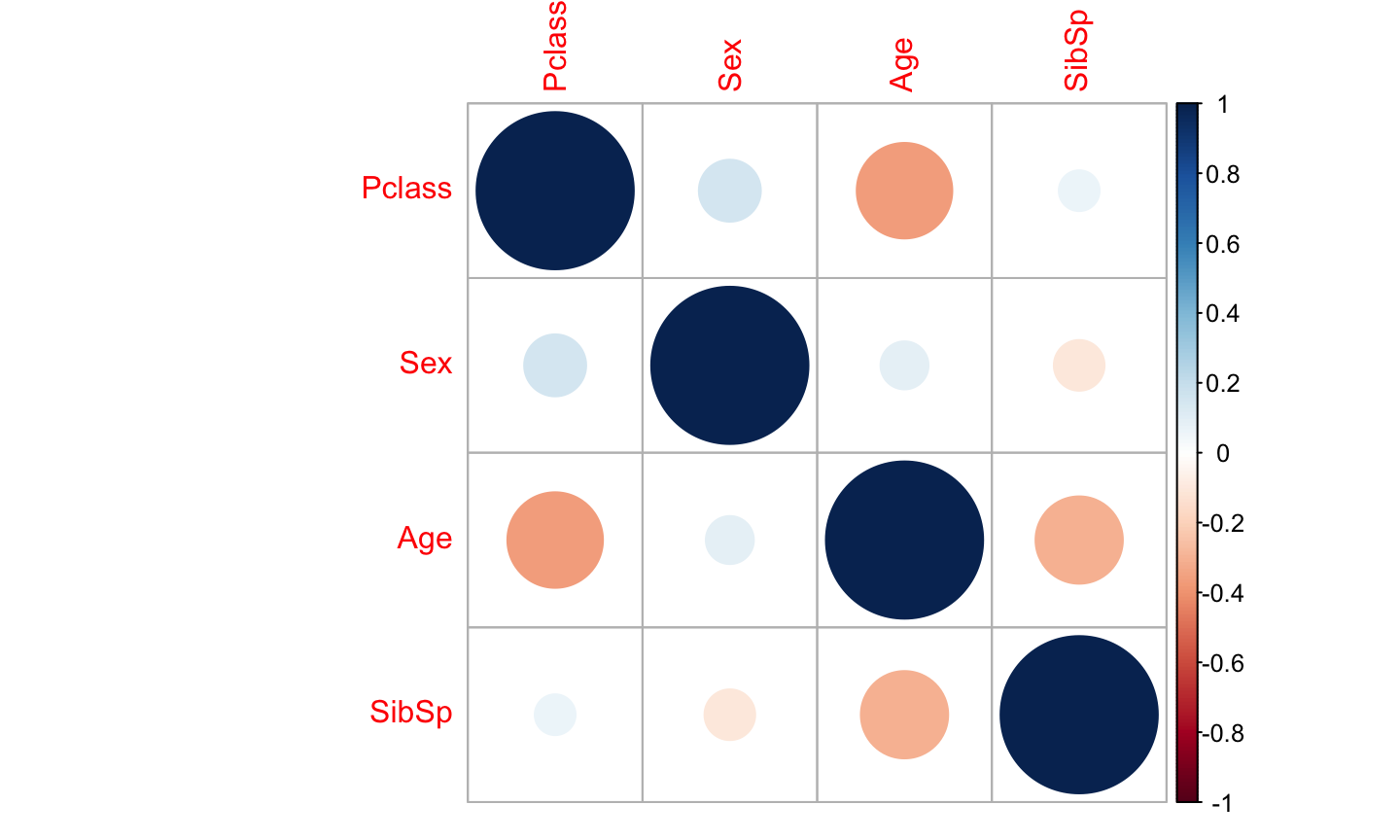## Build models ## Model evaluation methods ## 10-fold cross validation with 3 repeats trainControl <- trainControl(method = "repeatedcv", number = 10, repeats = 3) metric <- "Accuracy" ## logistic regression fit.glm <- train(Survived~., data=datasetTrain, method="glm", metric=metric, na.action = na.exclude, trControl=trainControl) ## KNN fit.knn <- train(Survived~., data=datasetTrain, method="knn", metric=metric, na.action = na.exclude, trControl=trainControl) ## Naive Bayes fit.nb <- train(Survived~., data=datasetTrain, method="nb", metric=metric, na.action = na.exclude, trControl=trainControl) ## Decision tree fit.cart <- train(Survived~., data=datasetTrain, method="rpart", metric=metric, na.action = na.exclude, trControl=trainControl) ## SVM fit.svm <- train(Survived~., data=datasetTrain, method="svmRadial", metric=metric, na.action = na.exclude, trControl=trainControl) ### Cross validation • The samples are randomly partitioned into k sets of roughly equal size. A model is fit using the all samples except the first subset (called the first fold). The held-out samples are predicted by this model and used to estimate performance measures. • The first subset is returned to the training set and procedure repeats with the second subset held out, and so on. • The k resampled estimates of performance are summarized and used to understand the relationship between the tuning parameter(s) and model utility. ## Algorithm comparison ## Compare algorithms results <- resamples(list(LG=fit.glm, KNN=fit.knn, CART=fit.cart, NB=fit.nb, SVM=fit.svm)) summary(results) ## ## Call: ## summary.resamples(object = results) ## ## Models: LG, KNN, CART, NB, SVM ## Number of resamples: 30 ## ## Accuracy ## Min. 1st Qu. Median Mean 3rd Qu. Max. NA's ## LG 0.7183099 0.7613948 0.7972418 0.7949661 0.8194444 0.8750000 0 ## KNN 0.5774648 0.6619718 0.6853482 0.6966354 0.7491197 0.7887324 0 ## CART 0.6666667 0.7473592 0.7777778 0.7754108 0.8028169 0.8611111 0 ## NB 0.6901408 0.7500000 0.7777778 0.7828638 0.8140649 0.8732394 0 ## SVM 0.7323944 0.8028169 0.8309859 0.8249218 0.8591549 0.9014085 0 ## ## Kappa ## Min. 1st Qu. Median Mean 3rd Qu. Max. NA's ## LG 0.41707718 0.5057304 0.5831935 0.5707940 0.6217023 0.7357259 0 ## KNN 0.07632264 0.2810573 0.3354720 0.3585996 0.4795261 0.5458422 0 ## CART 0.24804178 0.4487177 0.5335461 0.5182386 0.5802384 0.7080292 0 ## NB 0.37867940 0.4861467 0.5336617 0.5468811 0.6092111 0.7334168 0 ## SVM 0.43721318 0.5772053 0.6359640 0.6259419 0.6980581 0.7903838 0 dotplot(results)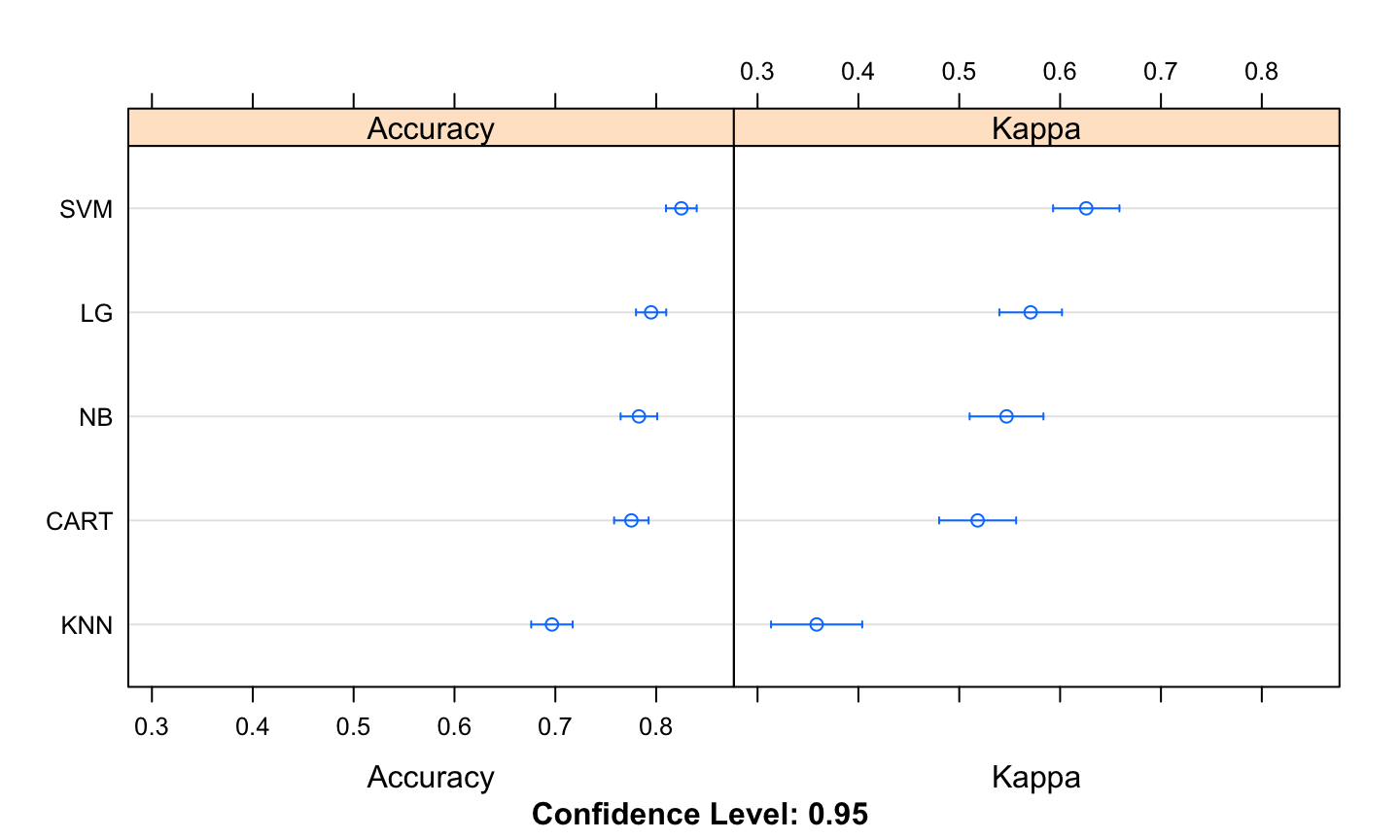## Finalize model model <- train(Survived~., data=datasetTrain, method="svmRadial", metric=metric, na.action = na.exclude, trControl=trainControl) testData <- datasetTest[,c(-1, -8, -10, -11)] testData$Age[is.na(testData$Age)] <- 0 testData$Fare[is.na(testData\$Fare)] <- 0
predictions <- predict(model, testData)